# Lesson Notes By Weeks and Term - Primary 3

2-D Shapes

TERM: 2nd Term

WEEK: 5

CLASS: Primary 3

AGE: 8 years

DURATION: 5 periods of 40 minutes each

DATE:

SUBJECT: Mathematics

TOPIC: 2-D shapes

SPECIFIC OBJECTIVES: At the end of the lesson, the pupils should be able to

1. Describe, sort and compare circles.
2. Describe, sort and compare triangles.
3. Describe, sort and compare squares.

INSTRUCTIONAL TECHNIQUES: Explanation, question and answer, demonstration, practical, assessments

INSTRUCTIONAL MATERIALS: String and sticks. scrap paper, advertisement flyers (collect and bring from home), videos from source-

PERIOD 1: Assessments

 PRESENTATION TEACHER’S ACTIVITY PUPIL’S ACTIVITY STEP 1ORALASSESSMENTS The teacher asks questions on time and calendars Pupils respond and participate STEP 2DISCUSSION The teacher discusses all the methods used by some learners in the oral assessments(some of the questions are solved on the board by the learners) and addresses any misconceptions that may have risen Pupils pay attention and participate STEP 3WRITTEN ASSESSMENTS 1 Write half past 7 in digital time.2 Write quarter to 9 in digital time.3 Circle the clock that shows quarter past two.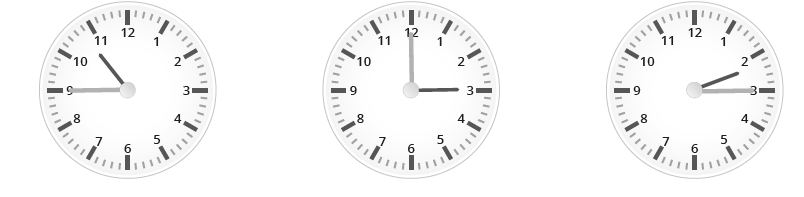4. show half past 3 in the afternoon on an analogue clock.5 Show quarter to 10 on an analogue and a digital clock.6 How much time passed between 2 o’clock and half past four in the afternoon? Pupils attempt their class work STEP 4SUMMARY The teacher marks the written assessments, corrects were necessary and commends the pupils

PERIOD 2: Circles

PRESENTATION

TEACHER’S ACTIVITY

PUPIL’S ACTIVITY

STEP 1

MENTAL MATHS

The teacher begins the lesson with some mental calculations

Calculate

1 5 × 10 =

2 2 × 10 =

3 7 × 10 =

4 1 × 10 =

5 4 × 10 =

6 3 × 10 =

7 10 × 10 =

8 0 × 10 =

9 6 × 10 =

10 8 × 10 =

Pupils respond and participate

STEP 2

CONCEPT

DEVELOPMENT

The teacher

• Describes a circle by saying, I am thinking about a shape. It is round and has no straight sides. What shape am I thinking about? (a circle)

• Ensures that you model the correct use of vocabulary.

CLASS ACTIVITY

The teacher

Takes the learners outside to a place where the learners can draw in the sand.

Lets the learners get into groups of 5.

- What shape did we discuss in class? ( a circle)

- What are the features of a circle? (It is round and has no straight sides.)

Instructs the class:

In your groups find a stick.

- Each of you draw a different size circle in the sand.

Asks: Who drew the biggest circle? Who drew the smallest circle?

Gives each group a piece of string.

Using the string make a circle.

- Which was easier to use to make a circle? A stick or string? Discuss this with your learners (no right answer here).

Returns to the classroom.

ACTIVITY II

The teacher ask the learners to

Draws a circle. Draw three more, but all of them should look different.

-Discuss with the class what is different about each one. (The differences could be in the

size of the shapes. Circles do not look different if their ‘orientation’ is changed.)

Pupils pay attention and participate

STEP 3

CLASS-WORK

1 Draw 3 different sized circles in the table below.

 Small circle Bigger circle Biggest circle

2. Draw circles in different positions in the table below.

 Circle at the top Circle in the middle Circle at the bottom

3 Use 6 circles to create a picture.

Pupils attempt their class work

STEP 4

HOME-WORK

Find and draw 3 objects that are circular in your home.

Pupils attempt their class work

STEP 5

SUMMARY

The tacher summarizes by reminding the pupils how to describe, compare and draw circles.

She marks their class works, makes corrections where necessary and commends them positively

PERIOD 3: Triangles

 PRESENTATION TEACHER’S ACTIVITY PUPIL’S ACTIVITY STEP 1MENTAL MATHS The teacher begins the lesson with some mental calculationsCalculate1 __ × 10 = 50  2 __ × 10 = 30  3 __ × 2 = 12  4 __ × 2 = 20  5 __ × 10 = 60  6 __ × 10 = 907 __ × 2 = 188 __ × 2 = 149 __ × 10 = 7010 __ × 2 = 0 Pupils respond and participate STEP 2CONCEPTDEVELOPMENT The teacher• Describes a triangle by saying, I am thinking about a shape. It has 3 straight sides and3 angles. What shape am I thinking about? (a triangle)• Ensures that you model the correct use of vocabulary. CLASS ACTIVITYThe teacher• Takes the learners outside to a place where the learners can draw in the sand.• Lets the learners get into groups of 5.- What shape did we discuss in class? ( a triangle)- What are the features of a triangle? (It has 3 straight sides and 3 angles)• Instructs the class:In your groups find a stick.Each of you draw a different size triangle in the sand.• Asks: Who drew the biggest triangle? Who drew the smallest triangle?• Gives each group a piece of string.Using the string make a triangle. Encourage the learners to use the correct vocabulary.Which was easier to use to make a triangle? A stick or string? Discuss this with your learners (no right answers here).• Returns to the classroom. ACTIVITY IIThe teacher ask the learners to- Draws a triangle. Draw three more, but all of them should look different.- Discuss with their partner about what is different about each one. (The differences could be inthe size and/or orientation of the shapes.) Pupils pay attention and participate STEP 3CLASS-WORK NOTE: Learners need to use scrap paper for question 1 of this activity.1 Use 2 square pieces of paper. Fold each one twice to make a square and a triangle.2 Stick them in your maths book and label them.3 Work in pairs. How many shapes do you see? Count very carefully.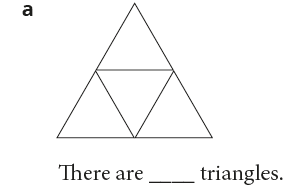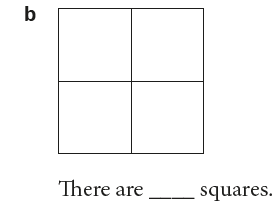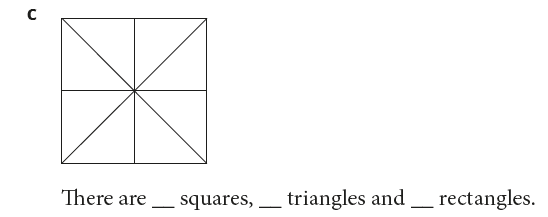2 Draw five triangles. They must all look different. 3 Find and cut triangles of different sizes from a magazine or newspaper. Stick them intoyour book, in different positions.a How many sides does each triangle have?b Are the sides straight or round? Pupils attempt their class work STEP 4HOME-WORK How many triangles are there in this picture?Pupils attempt their class work STEP 5SUMMARY The teacher summarizes by reminding the pupils how to draw, compare and discuss triangles.. She marks their class works, makes corrections where necessary and commends them positively

PERIOD 4: Squares

 PRESENTATION TEACHER’S ACTIVITY PUPIL’S ACTIVITY STEP 1MENTAL MATHS The teacher begins the lesson with some mental calculationsCalculate1 1 × 1 =  2 1 × 2 =  3 2 × 2 =  4 2 × 3 =  5 3 × 4 =  6 3 × 5 =7 3 x 6 =  8 4 × 5 =9 5 × 1 =10 10 × 2 = Pupils respond and participate STEP 2CONCEPTDEVELOPMENT The teacher• Describes a square by saying, I am thinking about a shape.It has 4 sides and 4 corners. All the sides are the same length and all the corners are the same size. What shape am I thinking about? (a square)• Ensures that you model the correct use of vocabulary. CLASS ACTIVITYThe teacher• Takes the learners outside to a place where the learners can draw in the sand.• Lets the learners get into groups of 5.What shape did we discuss in class? ( a square)- What are the features of a square? (It has 4 equal sides and 4 corners)• Instructs the class:In your groups find a stick.- Each of you draw a different size square in the sand.• Asks: Who drew the biggest square? Who drew the smallest square?• Gives each group a piece of string.- Using the string make a square. Encourage the learners to use the correct vocabulary.- Which was easier to use to make a square? A stick or string? Discuss this with your learners (no right answers here).• Returns to the classroom. Pupils pay attention and participate STEP 3CLASS-WORK 1 Draw a square. Draw three more, but all should look different.(Answers will vary. The differences could be in the size and/or orientation ofthe shapes.)2 Draw a picture that is made up of 8 different sized squares.3 Draw three squares:a A square with 4 cm sides.b A square with 7 cm sides.c A square with 10 cm sides. Pupils attempt their class work STEP 4HOME-WORK Find and draw 3 objects that are square in your home. Pupils attempt their class work STEP 5SUMMARY The teacher summarizes by reminding the pupils how to describe and compare squares.  She marks their class works, makes corrections where necessary and commends them positively

PERIOD 5: Weekly Test/consolidations

TEACHER’S ACTIVITY: The teacher revises all the concepts treated from period 1-4 and gives the pupils follow through exercises, quiz and tests . She marks the exercises, makes corrections and commends the pupils positively.

PUPIL’S ACTIVITY: The pupils work on the worksheets and exercises given by the teacher individually

CONSOLIDATION

1 Complete the table: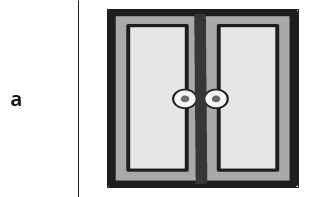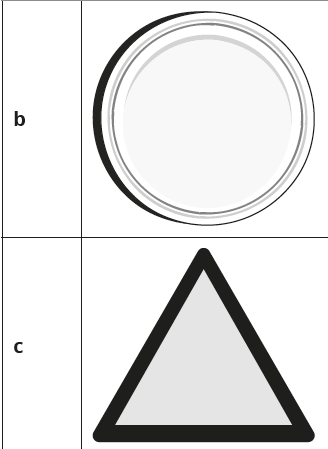2. Draw a car using triangles, circles and squares.